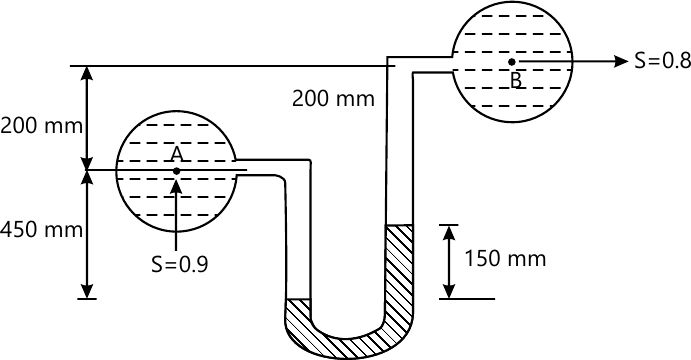VTU Mechanical Engineering (Semester 4)
Fluid Mechanics
June 2013
Total marks: --
Total time: --
INSTRUCTIONS
(1) Assume appropriate data and state your reasons
(2) Marks are given to the right of every question
(3) Draw neat diagrams wherever necessary

1 (a) Define the following properties of fluid:
Specific weight
specific gravity
Vapour pressure
Capability
Surface tension
10 M
1 (b) A shaft of 250mm diameter rotates inside a sleeve of 120mm long at 200rpm.If the thickness of oil film between shaft and sleeve is 1.5mm,find the power lost in friction.the dynamic viscosity of oil used is 8 poise
10 M

2 (a) obtained the total pressure and the centre of pressure on an inclined plain surface immersed in fluid
10 M
2 (b) A differential U -Tube mercury manometer is used to measure pressure between two water pipes A and B .Find the pressure at A if the level difference in mercury in the manometer is 150mm as shown fig.the pressure at B is 300 kPa10 M

3 (a) Explain the method to find metacentric height experimentally
8 M
3 (b) The stream function for a two-dimensional flow is given by?=2xy.Find the velocity at the point p(4,2). Also find the velocity potential function
12 M

4 (b) A 10km long water pipe is laid a slope of 3 in 4.The diameter of the lower end and upper end are 120mm and 180mm respectively pressure gauges fixed at the lower end upper end reads 0.2MPa and respectively.Determine the flow rate of water through the pipe
10 M
4 (a) Obtain the Euler\'s equation of motion along a stream line and hence derive Bernaulis equation for a steady incompressible fluid flow
10 M

5 (a) Derive an expression for discharge through a rectangular notch
10 M
5(b) Using Buckingham's π-theorem prove that the frictional torque T of a disc of diameter D rotating at a speed N in fluid of viscosity μ and density ρ in a turbulent flow is given by $T=D^{5}N^{2}\rho \phi \left [ \frac{M}{D^{2}N\rho } \right ]$
10 M

6 (a) Derive Darcy \'s equation for the loss of head due to friction in a circular pipe
10 M
6 (b) Water is supplied to a town having a population of 1 lakh from a reservoir 6km away from the town and is stipulated that half of the daily supply of 8-litres per head should be delivered in 8hrs .What should b e the diameter of the supplier pipe?The loss of head due to friction in the line is 2m.Take f=0.002
10 M

7 (a) Derive Hagen-Poiseuille\'s equation for viscous flow through a circular pipe
10 M
7 (b) A pipe of diameter 240mm and length is laid at a slope of 1 in 250 .An oil of specification gravity 0.85 and viscosity 180cp is pumped up at a rate of 10liters /sec. find the head lost due to friction and the power required to pump the oil
10 M

8 (a) Explain the following:
Drag
Lift
Momentum thickness
Mach number
Mach cone
10 M
8 (b) A flat plate 2m × 2m moves at 40km/hour in the stationary air density 1.2kg/m3If the coefficient of drag and lift are 0.75 respectively, determine
the lift force
the drag force
the resultant force
The power required to keep the plate in motion
10 M

More question papers from Fluid Mechanics